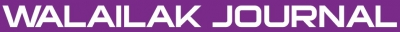### MHD Flow of a Non-Newtonian Power Law Fluid over a Vertical Stretching Sheet with the Convective Boundary Condition

#### Abstract

In this article, we study the power law model of steady state, viscous, incompressible MHD flow over a vertically stretching sheet. Furthermore, heat transfer is also addressed by using the convective boundary conditions. The coupled partial differential equations are transformed into ordinary differential equations (ODEs) using similarity transformations. The transformed highly non-linear ODEs are solved by using the Homotopy Analysis Method (HAM). The influence of different parameters on the velocity and temperature fields are analyzed and discussed.

#### Keywords

Power law model, heat transfer, convective boundary conditions, vertical stretching sheet

PDF

#### References

WR Schowalter. The application of boundary layer theory to power law pseudo-plastic: similar solutions. AIChE Journal 1960; 6, 24-8.

A Acrivos. A theoretical analysis of laminar natural convection heat transfer to non-Newtonian fluids. AIChE Journal 1960; 6; 584-90.

SY Lee and WF Amen. Similar solutions for non-Newtonian fluids. AIChE Journal 1966; 12, 700-8.

CJ Cran. Flow past a stretching plate. Zeitschrift für angewandte Mathematik und Physik 1970; 12, 645-7.

HI Anderson and BS Dandapat. Flow of a power law fluid over a stretching sheet. Stability Appl. Anal. Continuous Media 1991; 1, 339-47.

KV Parasad, PS Datti and K Vajravelu. Hydromagnetic flow and heat transfer of a non-Newtonian power law fluid over a vertical stretching sheet. Int. J. Heat and Mass Transf. 2010; 53, 879-88.

A Shahzad and R Ali. Approximate analytic solution for magneto-hydrodynamic flow of a non-Newtonian fluid over a vertical stretching sheet. Can. J. App. Sci. 2012; 2, 202-15.

D Makinde and A Aziz. Mixed convection from a convectively heated vertical plate to a fluid with internal heat generation. J. Heat Transfer 2011; 133, 122501.

WA Khan and I Pop. Flow and heat transfer over a continuously moving flat plate in a porous medium. J. Heat Transfer 2011; 133, 0504501.

VG Fox, LE Erickson and LE Fan. Methods for solving boundary layer equations for moving continuous flat surfaces with suction and injection. AIChE Journal 1969; 14, 726-36.

D Pal. Combined effects of non-uniform heat source / sink and thermal radiation on heat transfer over an unsteady stretching permeable surface. Commun. Nonlinear Sci. Numer. Simulat. 2011; 16, 1890-904.

T Hayat, H Zaman and M Ayub. Analytical solution of hydromagnetic flow with Hall effect over a surface stretching with a power-law velocity. Num. Math. Partial Diff. Eqn. 2011; 27, 937-59.

IA Hassanien, AA Abdullah and RSR Gorla. Flow and heat transfer in a power law fluid over a non-isothermal stretching sheet. Math. Comput. Model. 1998; 9, 105-16.

HI Anderson, KH Bech and BS Dandapat. Magnetohydrodynamic flow of a power-law fluid over a stretching sheet. Int. J. Non-Linear Mech. 1992; 27, 929-36.

KV Parasad and K Vajravelu. Heat transfer in the MHD flow of a power law fluid over a non-isothermal stretching sheet. Int. J. Heat Mass Transf. 2009; 52, 4956-65.

A Aziz. A similarity solution for laminar thermal boundary layer flow over a flat plate with a convective surface boundary condition. Commun. Nonlinear Sci. Numer. Simulat. 2009; 14, 1064-8.

R Cortell. Suction, viscous dissipation and thermal radiation effects on the flow and heat transfer of a power-law fluid past an infinite porous plate. Chem. Eng. Res. Des. 2010; 85, 85-93.

SJ Liao. A new branch of solutions of boundary layer flows over an incompressible stretching plate. Int. J. Heat Mass Transf. 2005; 48, 2529-39.

SJ Liao. A short review on the homotopy analysis method in fluid mechanics. J. Hydrodynamics 2010; 22, 882-4.

T Hayat, M Khan and M Ayub. On the explicit analytic solutions of an Oldroyd 6-constant fluid. Int. J. Eng. Sci. 2004; 42, 123-35.

TR Mahapatra, SK Nandy and AS Gupta. Analytic solution of magnetohydrodynamic stagnation-point flow of a power-law fluid towards a stretching surface. Appl. Math. Comput. 2009; 215, 1696-710.

S Abbasbandy. Homotopy analysis method for the Kawahara equation. Nonlinear Anal. Real World Appl. 2010; 11, 307-12.

PK Rao, AK Sahu and RP Chhabra. Momentum and heat transfer from a square cylinder in power law fluids. Int. J. Heat Mass Transf. 2011; 54, 390-403.

### Refbacks

• There are currently no refbacks.Online ISSN: 2228-835X

http://wjst.wu.ac.th

Last updated: 20 June 2019